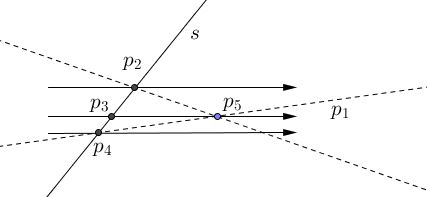# Sylvester's Problem, Gallai's Solution

The Sylvester Problem has been posed by James Joseph Sylvester in 1893 in Educational Times:

Let $n$ given points have the property that the line joining any two of them passes through a third point of the set. Must the $n$ points all lie on one line?

T. Gallai's proof has been outlined by P. Erdös in his submission of the problem to The American Mathematical Monthly in 1943.

### Solution

Given the set $\Pi$ of noncollinear points, consider the set of lines $\Sigma$ that pass through at least two points of $\Pi.$ Such lines are said to be connecting. Among the connecting lines, those that pass through exactly two points of $\Pi$ are called ordinary.

Choose any point $p_{1}\in\Pi.$ If $p_{1}$ lies on an ordinary line we are done, so we may assume that $p_{1}$ lies on no ordinary line. Project $p_{1}$ to infinity and consider the set of connecting lines containing $p_{1}.$ These lines are all parallel to each other, and each contains $p_{1}$ and at least two other points of $\Pi.$ Any connecting line not through $p_{1}$ forms an angle with the parallel lines; let $s$ be a connecting line (not through $p_{1})$ which forms the smallest such angle:Then $s$ must be ordinary! For suppose $s$ were to contain three (or more) points of $\Pi,$ say, $p_{2},$ $p_{3},$ $p_{4}$ named so that $p_{3}$ is between $p_{2}$ and $p_{4}:$The connecting line through $p_{3}$ and $p_{1}$ (being not ordinary) would contain a third point of $\Pi,$ say $p_{5},$ and now either the line $p_{2}p_{5}$ or the line $p_{4}p_{5}$ would form a smaller angle with the parallel lines than does $s.$

### References

1. P. Borwein, W. O. J. Moser, A survey of Sylvester's problem and its generalizations, Aequationes Mathematicae 40 (1990) 111 - 135
2. P. Erdös, R. Steinberg, Problem 4065 [1943, 65], The American Mathematical Monthly, Vol. 51, No. 3 (Mar., 1944), pp. 169-171
3. J. J. Sylvester, Educational Times, Mathematical Question 11851, vol. 59 (1893), p. 98Copyright © 1996-2018 Alexander Bogomolny

 65264580